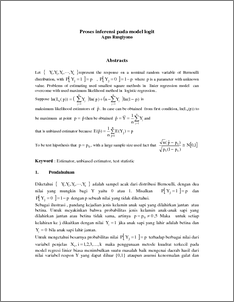# Proses inferensi pada model logit

Rusgiyono, Agus (2008) Proses inferensi pada model logit. Media Statistika, 1 (2). pp. 89-96. ISSN 1979-3693Preview
PDF
142Kb

## Abstract

Let represent the response on a nominal random variable of Bernoulli distribution, with , where is a parameter with unknown value. Problems of estimating used smallest square methods in linier regression model can overcome with used maximum likelihood method in logistic regression.. Suppose is maksimum likelihood estimstors of . In case can be obtained from first condition, ln(Ln(p)) to be maximum at point then be obtained and that is unbiased estimator because To be test hipothesis that , with a large sample size used fact that Keyword : Estimator, unbiased estimator, test statistic

Item Type: Article Q Science > Q Science (General) Faculty of Science and Mathematics > Department of Statistics 32675 INVALID USER 30 Jan 2012 10:07 30 Jan 2012 10:07

Repository Staff Only: item control page